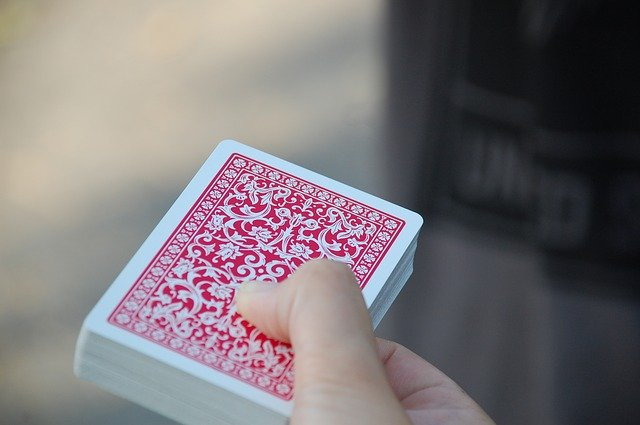## 16.5 Independence

One important concept in probability is independence. Two events are independent if the probability of one event happening is the same, whether or not the other event has happened.

For example, if you toss a coin, the probability of getting a head is the same whether you are sitting or standing. That is, the result of a coin toss is independent of your position.Definition 16.7 (Independence) Two events are independent if the probability of one event is the same, whether or not the other event has happened.Example 16.10 (Independence) Consider drawing two cards from a fair pack (of 52 cards), without returning the first card.

For the first card, the sample space lists every card in the pack, and drawing any one card is as equally likely as drawing any other. Since four cards are Aces, the probability of drawing an Ace on the first draw is 4/52 (using the classical approach to probability).

If we drew an Ace for the first card, the probability of drawing an Ace for the second card is 3/51 (three Aces remain among the 51 remaining cards).

Alternatively, if we don’t draw an Ace for the first card, the probability of drawing an Ace second time is 4/51 (four Aces remain among the 51 remaining cards).

In summary, the probability of drawing an Ace for the second card depends on whether or not an Ace was drawn for the first card. The two events ‘Drawing an Ace for the first card’ and ‘Drawing an Ace for the second card’ are not independent events.